## MATHS :: Lecture 10 :: INTEGRATION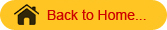INTEGRATION
Integration is a process, which is a inverse of differentiation. As the symbol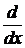represents differentiation with respect to x, the symbol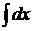stands for integration with respect to x.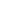Definition
If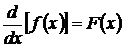then f(x) is called the integral of F(x) denoted by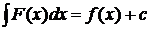. This can be read it as integral of F(x) with respect to x is f(x) + c where c is an arbitrary constant. The integral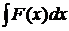is known as Indefinite integral and the function F(x) as integrand.

Integration by parts Examples I

Integration by parts Examples II

Integration by parts Examples III

Formula on integration
1).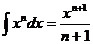+c  ( n ¹-1)
2).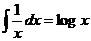+c
3).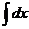=  x+c
4).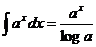+c
5).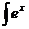dx = ex +c
6).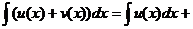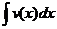7).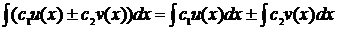8).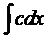= c x + d
9).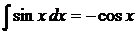+c
10).+c
11).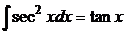+c
12).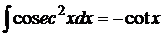+c
13).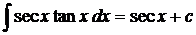14).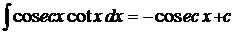13).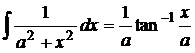+c
14).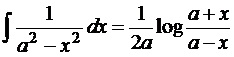+c

15).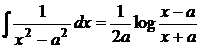+c
16).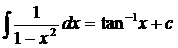Definite integral
If  f(x)  is indefinite integral of F(x) with respect to x then the Integral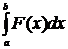is called definite integral of F(x) with respect to x from x = a to x = b. Here a is called the Lower limit and b is called the Upper limit of the integral.=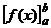=  f(Upper limit ) - f(Lower limit)
=  f(b) - f(a)
Note
While evaluating a definite integral no constant of integration is to be added. That is a definite integral has a definite value.

###### Method of substitution

Method –1
Formulae for the functions involving (ax + b)
Consider the integral
I =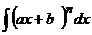-------------(1)
Where a and b are constants
Put a x + b = y
Differentiating with respect to x
a dx + 0 = dy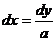Substituting in (1)
I =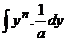+c
=+c
=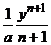+c
=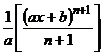+ c
Similarly this method can be applied for other formulae also.

Method II
Integrals of the functions of the form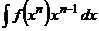put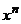=y,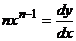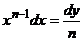Substituting we get
I =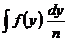and this can be integrated.
Method –III
Integrals of function of the type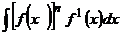when n ¹ -1, put f(x) = y then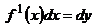\=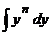=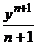=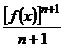when n= -1, the integral reduces to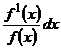putting y = f(x) then dy = f1(x) dx
\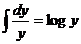=log f(x)
Method IV

# Method of Partial Fractions

Integrals of the form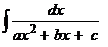Case.1
If denominator can be factorized into linear factors then we write the integrand as
the sum or difference of  two linear factors of the form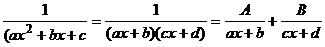###### Case-2

In the given   integralthe denominator ax2 + bx + c can not be factorized into linear factors, then express ax2 + bx + c as the sum or difference of two perfect squares and then apply the formulae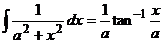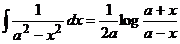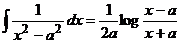Integrals of the form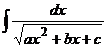Write denominator as the sum or difference of two perfect squares=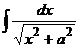or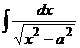or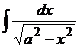and then apply the formula= log(x+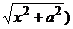= log(x+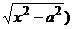Integration by parts
If the given integral is of the form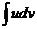then this can not be solved by any of techniques studied so  far. To solve this  integral we first take the product rule on differentiation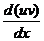=u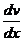+v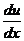Integrating both sides we get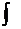dx=( u+v)dx
then we have    u v=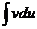+re arranging the terms we   get= uv-This formula is known as integration by parts formula
Select the functions u and dv appropriately in such a way that integralcan be more easily integrable than the given integral

Application of integration
The area bounded by the function y=f(x), x=axis and the ordinates at x=a x=b is given by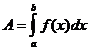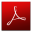Download this lecture as PDF here